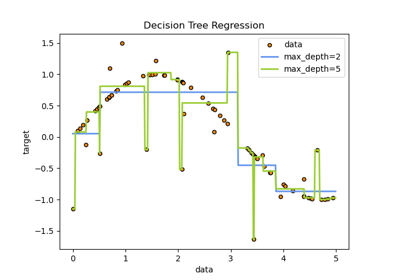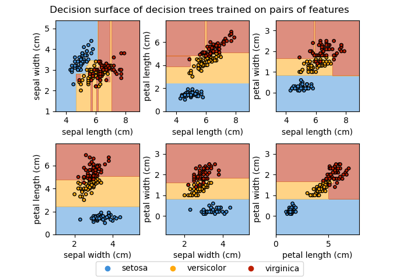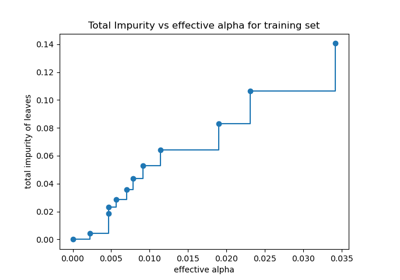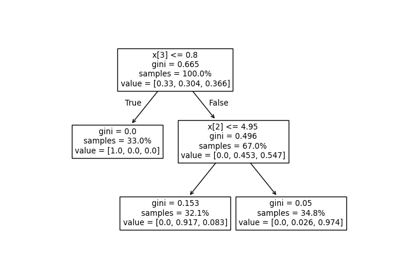# Decision Trees¶

Examples concerning the sklearn.tree module.Decision Tree Regression

Decision Tree RegressionMulti-output Decision Tree Regression

Multi-output Decision Tree RegressionPlot the decision surface of decision trees trained on the iris dataset

Plot the decision surface of decision trees trained on the iris datasetPost pruning decision trees with cost complexity pruning

Post pruning decision trees with cost complexity pruningUnderstanding the decision tree structure

Understanding the decision tree structure